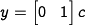# Errata: In equation (3.27), the state used for the output should be c, not x

The output in equation (3.27) usesas the state variable instead of. The correct output equation is